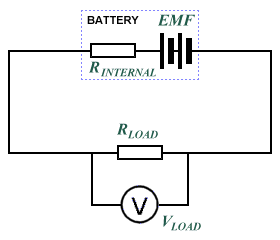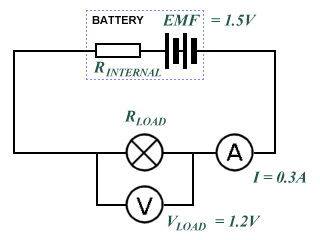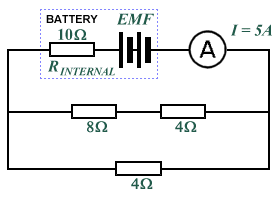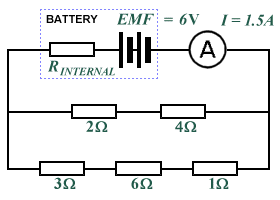# Internal Resistance

Any source of electrical energy such as a battery or a generator supplies electrical power by converting some other form of energy to electrical energy. In the case of a battery, it is the energy created by a chemical reaction of some kind that is used. The battery provides an electro-motive force (EMF) that pushes electrons around any electrical circuit to which it is connected. This electro-motive force can be expressed as a voltage, and is defined as the total amount of energy (in joules) per unit charge (in coulombs) supplied to the circuit. It can be represented using the following formula:

 ℰ  = E Q

where:

E = the energy in joules

Q = the charge in coulombs

= the electro-motive force

The potential difference (pd) across each component in the circuit is also expressed as a voltage, but is defined as the energy per unit charge converted to other forms of energy by the component. The total emf provided by the battery is essentially the open circuit voltage of the battery, i.e. the voltage measured when there is no load connected to the battery, and no current is flowing. In practical terms, this can be measured reasonably accurately with a modern digital multimeter, since the amount of current drawn by the multimeter can be regarded as insignificant. In reality, the potential difference measured across the terminals of a battery when it is connected to a load will be less than its open circuit voltage. The reason for this is that a battery is not an ideal voltage source, because as well as driving current through the load, the battery must also drive current through its own internal resistance, which will cause it to dissipate power as heat.

The internal resistance of a chemical battery is typically between a fraction of an ohm and a few ohms, and is mainly due to the resistance of the electrolytic materials used in the manufacture of the battery. Current must flow between the battery's electrodes and through these materials when the battery is connected to an electrical circuit. A voltage source such as a battery can therefore be seen as an ideal voltage source (one with no internal resistance) in series with a resistor (the battery's internal resistance). When current flows through the battery, there will be a small voltage drop across the internal resistance. This voltage drop can be calculated as the current multiplied by the internal resistance (Ohm's law). When a load is applied to the voltage source, the voltage drop across the load resistance (the terminal voltage) will be equal to the source emf minus the voltage drop across the internal resistance, since the two resistances are in series with one another. The diagram below illustrates the principle.The battery's internal resistance is in series with the load resistance

The internal resistance of a battery will vary according to the battery type (i.e. alkaline, lead-acid, nickel-cadmium etc.), and may vary with load, temperature, and the age of the battery. Disposable batteries supply less voltage over time, for example, because their internal resistance steadily increases. Eventually, the voltage produced will be so small that the battery is of no practical used and must be discarded. If the emf () of a battery is known, its internal resistance ( RINTERNAL ) can be found by measuring the current ( I LOAD ) that flows across a load resistance ( RLOAD ) with a known value. Since the internal and load resistances are in series, we can use Kirchhoff's voltage law and Ohm's law to obtain the following formula:

Solving for RINTERNAL, we get:

Consider the circuit shown below, in which a battery with a known emf of 1.5 V is connected in series with a lamp. The voltage drop measured across the lamp VLOAD is 1.2 V, which means that we have "lost" 0.3 V across the internal resistance RINTERNAL. The resistance of the wires in the circuit can be considered to be negligible, while the resistance of the lamp RLOAD is unknown. The current I flowing through the circuit is measured as 0.30 A. How do we find the internal resistance?What is the internal resistance of the battery?

Since we know that R = V/I (Ohm's law) we can find the load resistance as follows:

 RLOAD  = VLOAD = 1.2 V =  4 Ω I 0.3 A

Now, using the formula we previously obtained for internal resistance:

 RINTERNAL  = 1.5 V -  4 Ω  =  1 Ω 0.3 A

The internal resistance of a source of electrical energy is an important factor when considering how to get the source to deliver maximum power to an electrical appliance (the load) connected to it. Although we will be looking at the subject of power in more detail elsewhere, the maximum power theorem asserts that maximum power transfer occurs when the internal resistance of the source is equal to the load resistance. The down side to this is that the power dissipated in the source itself is also equal to the power transferred to the load (Power = I 2 × R), giving a power efficiency of only 50%. The most efficient transfer of power takes place when the external (load) resistance is much greater than the internal resistance of the source. The needs of the application must be carefully considered, therefore, when deciding on the best type of source to use. A lead-acid car battery, for example, is required to deliver relatively high currents at a comparatively low voltage (most car batteries supply a nominal emf of 12.6 volts). Its low internal resistance allows it to provide these high currents without its terminal voltage falling significantly. High-voltage power supplies, on the other hand, must have an extremely high internal resistance in order to limit the amount of current that would flow in the event of an accidental short-circuit.

## Problems

1. A 9 V battery is connected in series with a load and the terminal voltage is found to be 8 V. Current through the circuit is measured as 5 A. What is the internal resistance of the battery?

2. What is the EMF of the battery in the following circuit?3. What is the internal resistance of the battery in the following circuit?4. A battery has an internal resistance of 0.5 Ω and an EMF of 1.5 V. When connected in series to a load resistance, the terminal voltage falls to 1.45 V. What current is flowing in the circuit, and what is the value of the load resistance?

5. What is the terminal voltage for a battery with an emf of 12 V and an internal resistance of 0.5 Ω when it is connected in series with to a 10 Ω resistor?